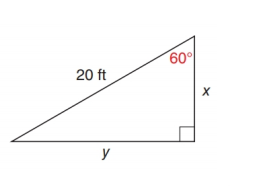Chapter 11.2, Problem 20E### Elementary Geometry for College St...

6th Edition
Daniel C. Alexander + 1 other
ISBN: 9781285195698

#### Solutions

Chapter
Section### Elementary Geometry for College St...

6th Edition
Daniel C. Alexander + 1 other
ISBN: 9781285195698
Textbook Problem
1 views

# In Exercise 17 to 22, use either the sine ratio or the cosine ratio to find the length of the indicated sides of the triangle correct to the nearest tenth of a unit.To determine

To find:

The length of the indicated sides of the following triangle,Explanation

Consider the following figure,

General formula for cosine ratio and sin ratio is given below,

From the given figure the value 20ft is the hypotenuse value since it is opposite to right angle.

Then x. is the length of the leg adjacent to 60 and y is the length of leg opposite to 60.

Therefore,

cos60=x20x=20×cos60

Using a scientific calculator in degree mode, follow the following key sequence,

60cos0

### Still sussing out bartleby?

Check out a sample textbook solution.

See a sample solution

#### The Solution to Your Study Problems

Bartleby provides explanations to thousands of textbook problems written by our experts, many with advanced degrees!

Get Started

#### Is 460 divisible by3?

Elementary Technical Mathematics

#### What is the lowest score in the following distribution?

Essentials of Statistics for The Behavioral Sciences (MindTap Course List)

#### ∫(x − cos x)dx = 1 − sin x + C 1 + sin x + C

Study Guide for Stewart's Single Variable Calculus: Early Transcendentals, 8th